Position, Rotation and Scaling

The 101 course only considers the setting of a mesh's position, rotation, and scaling. Further Reading will show you a range of methods to translate and rotate a mesh by a given amount.

Whatever the methods used they require a frame of reference, a means to describe the position, rotation or scaling and something to help visualize the effects of applying these. The visualization will be helped by the Pilot a constructed asymmetric shape.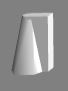The Pilot

Frames of Reference

There are two frames of reference that Babylon.js uses, the world axes and the local axes. The origin of the world axes never changes.

In all diagrams and playgrounds X axis is red, Y axis is Green and Z axis is blue.

When meshes are created their center is placed at the origin of the world axes and their position is always placed relative to the world axes.

The local axes move with the mesh. The origin of local axes is always at the created center of the mesh whatever its position. The centers of rotation and enlargement for a mesh are at the origin of the local axes, however by using either a TransformNode or a matrix to set a pivot point they can be changed to that point.

Vectors

All positions, rotations and scaling are given as 3 dimensional vectors using new BABYLON.Vector3(x, y, z) and can be set separately.

The Pilot

Following its creation the pilot's position is at the world origin with rotation zero for all axes and its scaling is one, both world axes and the pilot's local axes coincide.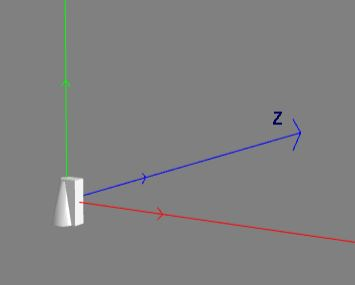Position

Position places the pilot with reference to the world axes using a vector (x, y, z). The local axes move with the pilot.

pilot.position = new BABYLON.Vector3(2, 3, 4);

or individual components

pilot.position.x  =  2;
pilot.position.y  =  3;
pilot.position.z  =  4;

The local and world axes remain in the same orientation.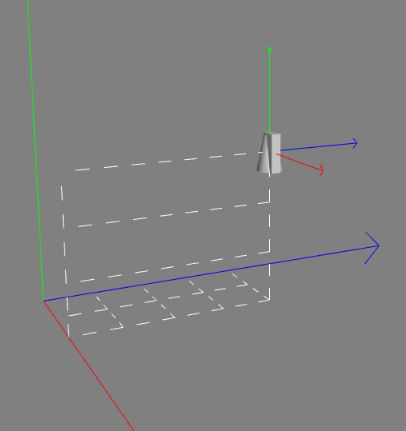Rotation

WARNING: Rotating in 3D space is always tricky. The order in which rotations are applied to a shape changes the final orientation of the shape and you also need to know which frame of reference is being used. There are many varying conventions for applying rotations in 3D modelling. For more details on these conventions in Babylon.js see Applying Rotations Convention BJS.

In Babylon.js rotations are set using

pilot.rotation = new BABYLON.Vector3(alpha, beta, gamma);

or

pilot.rotation.x  =  alpha; //rotation around x axis
pilot.rotation.y  =  beta;  //rotation around y axis
pilot.rotation.z  =  gamma; //rotation around z axis

where alpha, beta, and gamma are angles measured in radians.

PAUSE FOR THOUGHT: Immediately, since three rotations are given about three different axes you need to ask - "in which order are they applied about which frames of reference and in which direction?"

Either of the following two conventions can be considered as being used by rotation in Babylon.js since both lead to the same outcome.

Convention 1 - Local Axes

For local axes using rotation turns the mesh with the center of rotation at the origin of the local axes in the axes order y, x, z about the local axes. All rotations are anticlockwise when looking in the direction of the positive axis.

The following sequence of images shows the initial starting position of the pilot followed by a rotation of π/2 about the local y axis, then π/2 about the local x axis and finally a rotation of π/2 about the local z axis.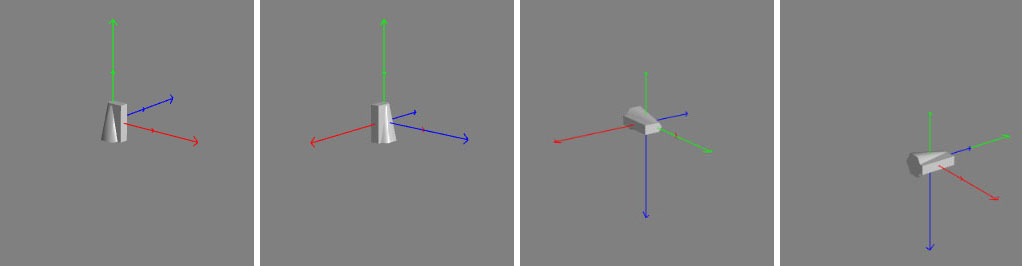The smaller axes represent the direction of the world axes.

Convention 2 - World Axes

Compared to convention 1 the center of rotation does not change but the axes of rotation do.

For world axes using rotation turns the mesh with the centre of rotation at the origin of the local axes in the axes order z, x, y about axes parallel to the world axes. All rotations are anticlockwise when looking in the direction of the positive axis.

The following sequence of images shows the initial starting position of the pilot followed by a rotation of π/2 about the world z axis, then π/2 about the world x axis and finally a rotation of π/2 about the world y axis.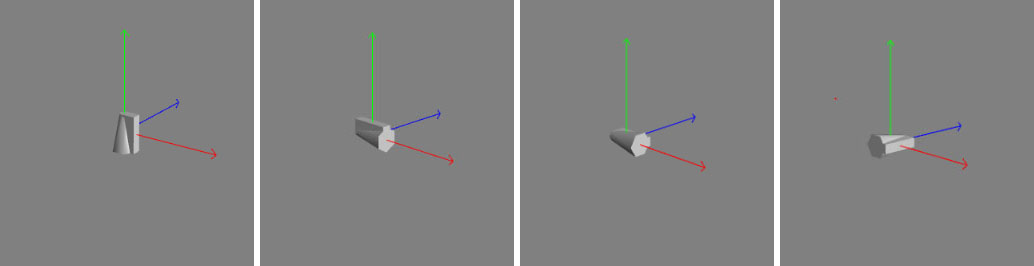Summary

For rotation whichever way you think about it the results are always the same. All the following commands produce the same outcome:

pilot.rotation = new BABYLON.Vector3(alpha, beta, gamma);

pilot.rotation.x  =  alpha;
pilot.rotation.y  =  beta;
pilot.rotation.z  =  gamma;

pilot.rotation.z  =  gamma;
pilot.rotation.x  =  alpha;
pilot.rotation.y  =  beta;

pilot.rotation.y  =  beta;
pilot.rotation.z  =  gamma;
pilot.rotation.x  =  alpha;

Sequencing Rotations

The question now is, what to do if you want a sequence of rotations that starts with one about the x axis, then about the y axis then about the z axis?

For world axes you use the rotate method. For rotations about local axes, Babylon.js has both the rotate method and addRotation method.

You can chain a sequence of rotations using the addRotation. This method provides for one rotation value about one axis a series of which can be applied from the first to the last as the following example shows.

The following sequence of images shows the result of adding the individual rotations one after the other for the above playground, starting with the initial position of the pilot followed by a rotation of π/2 about the local x axis, then π/2 about the local y axis and finally a rotation of π/2 about the local z axis.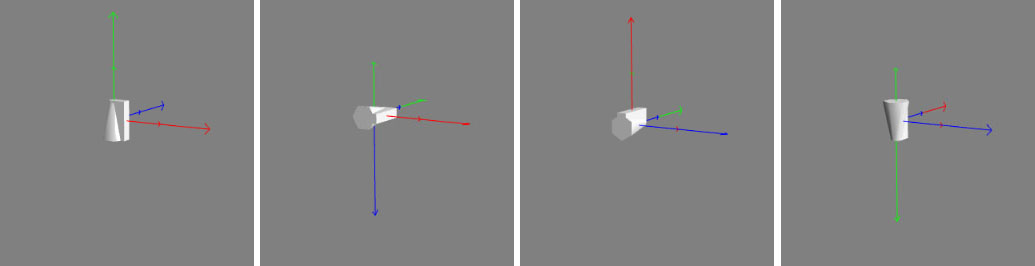The smaller axes represent the direction of the world axes.

In general mesh.addRotation(alpha, beta, gamma) needs at least two of alpha, beta, gamma to be 0 where alpha is a rotation about the local x axis, beta about the local y axis and gamma about the local z axis.

RotationQuaternions

An alternative to _rotations are rotationQuaternions though they can be tricky to use but can overcome some gimbal lock problems. Using both on a mesh is not possible see warning

Scaling

Scaling along the x, y, and z local axes is set using

mesh.scaling = new BABYLON.Vector3(scale_x, scale_y, scale_z);

or individually with

mesh.scaling.y = 5;

The following image shows a unit cube rotated about the z axis and scaled along the local y axis.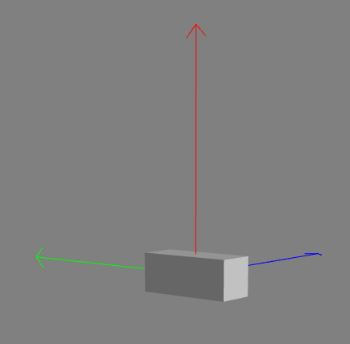Next step

Now you know how to create and move objects in a scene, but all your meshes have the same 'skin'. Not for long, if you read our next tutorial about materials.